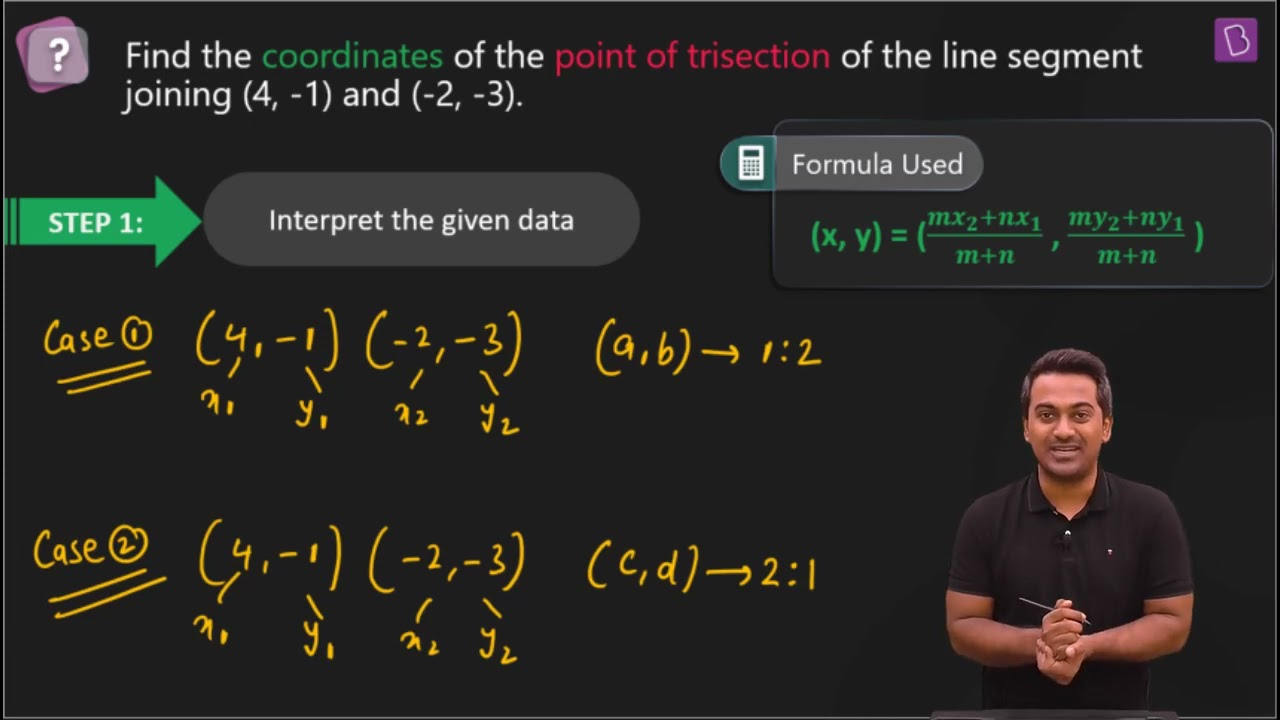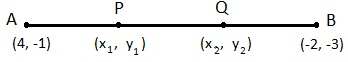Find the coordinates of the points of trisection of the line segment joining (4, –1) and (–2, –3).Let P (x1, y1) and Q (x2, y2) are the points of trisection of the line segment joining the given points i.e., AP = PQ = QB

Therefore, point P divides AB internally in the ratio 1:2.

x1 = (1×(-2) + 2×4)/3 = (-2 + 8)/3 = 6/3 = 2

x1 = 2

y1 = (1×(-3) + 2×(-1))/(1 + 2) = (-3 – 2)/3 = -5/3

y1 = -5/3

Therefore: P (x1, y1) = P(2, -5/3)

Point Q divides AB internally in the ratio 2:1.

x2 = (2×(-2) + 1×4)/(2 + 1) = (-4 + 4)/3 = 0

y2 = (2×(-3) + 1×(-1))/(2 + 1) = (-6 – 1)/3 = -7/3

The coordinates of the point Q is (0, -7/3)(2)(0)